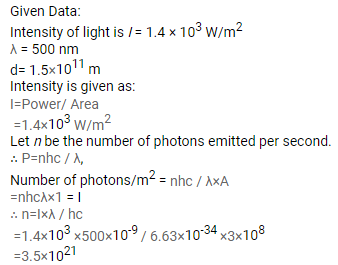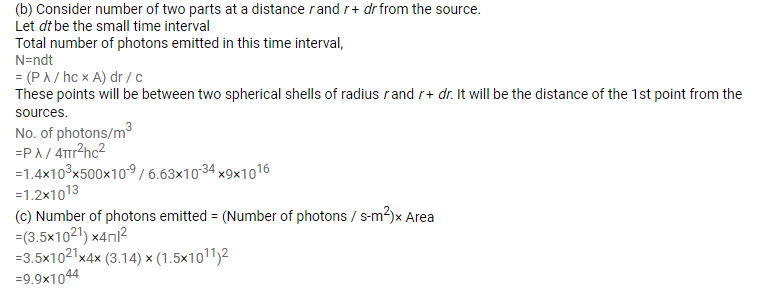# When the sun is directly overhead,

Question:

When the sun is directly overhead, the surface of the earth receives $1.4 \times 10^{3} \mathrm{~W} \mathrm{~m}^{-2}$ of sunlight. Assume that the light is monochromatic with average wavelength $500 \mathrm{~nm}$ and that no light is absorbed in between the sun and the earth's surface. The distance between the sun and the earth is $1.5 \times 10^{11} \mathrm{~m}$.

(a) Calculate the number of photons falling per second on each square metre of earth's surface directly below the sun.

(b) How many photons are there in each cubic metre near the earth's surface at any instant?

(c) How many photons does the sun emit per second?

Solution: